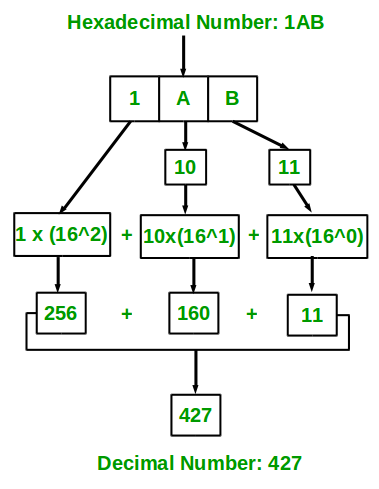Java Program For Hexadecimal to Decimal Conversion

• Last Updated : 09 Mar, 2021

Given a Hexadecimal number N, convert N into an equivalent decimal number i.e convert the number with base value 16 to base value 10. The decimal number system uses 10 digits 0-9 and the Hexadecimal number system uses 0-9, A-F to represent any numeric value.

Illustration:

Input : 1AB
Output: 427

Input : 1A
Output: 26

Approach:

1. The idea is to extract the digits of a given hexadecimal number starting from the rightmost digit.
2. Keep a variable ‘dec_value’.
3. At the time of extracting digits from the hexadecimal number, multiply the digit with the proper base (Power of 16) and add it to the above variable taken that is ‘dec_value’.
4. In the end, the variable ‘dec_value’ will store the required decimal number.convert hexadecimal number ( 1AB ) to equivalent decimal value

Implementation:

Example

Java

 // Java program to convert Hexadecimal to Decimal Number    // Importing input output classes import java.io.*;    // Main class class GFG {        // Method     // To convert hexadecimal to decimal     static int hexadecimalToDecimal(String hexVal)     {         // Storing the length of the         int len = hexVal.length();            // Initializing base value to 1, i.e 16^0         int base = 1;            // Initially declaring and initializing         // decimal value to zero         int dec_val = 0;            // Extracting characters as         // digits from last character            for (int i = len - 1; i >= 0; i--) {                // Condition check             // Case 1             // If character lies in '0'-'9', converting             // it to integral 0-9 by subtracting 48 from             // ASCII value             if (hexVal.charAt(i) >= '0'                 && hexVal.charAt(i) <= '9') {                 dec_val += (hexVal.charAt(i) - 48) * base;                    // Incrementing base by power                 base = base * 16;             }                // Case 2             // if case 1 is bypassed                // Now, if character lies in 'A'-'F' ,             // converting it to integral 10 - 15 by             // subtracting 55 from ASCII value             else if (hexVal.charAt(i) >= 'A'                      && hexVal.charAt(i) <= 'F') {                 dec_val += (hexVal.charAt(i) - 55) * base;                    // Incrementing base by power                 base = base * 16;             }         }            // Returning the decimal value         return dec_val;     }        // Method 2     // Main driver method     public static void main(String[] args)     {         // Custom input hexadecimal number to be         // converted into decimal number         String hexNum = "1A";            // Calling the above method to convert and         // alongside printing the hexadecimal number         System.out.println(hexadecimalToDecimal(hexNum));     } }

Output

26

Attention reader! Don’t stop learning now. Get hold of all the important Java Foundation and Collections concepts with the Fundamentals of Java and Java Collections Course at a student-friendly price and become industry ready. To complete your preparation from learning a language to DS Algo and many more,  please refer Complete Interview Preparation Course.

My Personal Notes arrow_drop_up
Recommended Articles
Page :